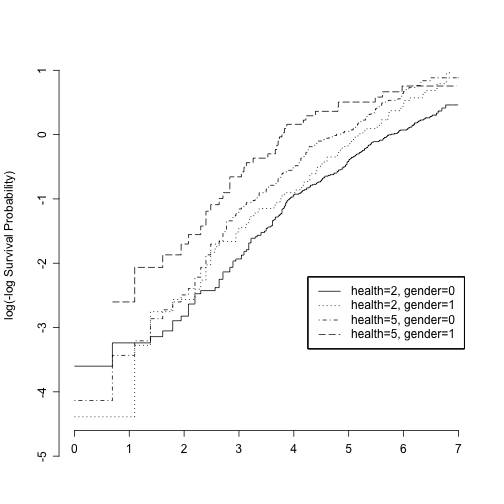# BIO 223 Applied Survival Analysis: Checking model fit and poroportional hazard assupmtion

## References

## Load halibut fishing dataset
col.names = c("id","survtime","censor","towdur","depth","length","handling","logcatch"))


## Fit Cox regression

## Load survival package
library(survival)

## Create survival vector for fish dataset
fish$SurvObj <- with(fish, Surv(survtime, censor == 1)) ## Fit Cox regression res.coxph <- coxph(SurvObj ~ towdur + handling + length + logcatch, data = fish) ## Extract linear predictor ## lp is for linear predictor = log(HR), relative to overall means by default fish$lp <- predict(res.coxph, type = "lp", reference = "sample")
##
## The Cox model is a _relative_ risk model; predictions of type
## "linear predictor", "risk", and "terms" are all relative to the
## sample from which they came.  By default, the reference value for
## each of these is the mean covariate within strata.  The primary
## underlying reason is statistical: a Cox model only predicts
## relative risks between pairs of subjects within the same strata,
## and hence the addition of a constant to any covariate, either
## overall or only within a particular stratum, has no effect on the
## fitted results. Using the ‘reference="strata"’ option causes this
## to be true for predictions as well.
##
## When the results of ‘predict’ are used in further calculations it
## may be desirable to use a fixed reference level. Use of
## ‘reference="sample"’ will use the overall means, and agrees with
## the ‘linear.predictors’ component of the coxph object (which uses
## the overall mean for backwards compatability with older code).
##
## Predictions of ‘type="terms"’ are almost invariably passed forward
## to further calculation, so for these we default to using the
## sample as the reference.


## Martingale Residuals

Martingale residuals are defined for the i-th individual as:

$$r_i = \delta_i - \hat{\Lambda}(T_i)$$

where $$\delta_i$$ is the number of event for the i-th subject between time 0 and $$T_i$$, and $$\hat{\Lambda}(T_i)$$ is the expected numbers based on the fitted model.

Properties:

• Mean = 0
• Range = [-$$\infty$$, 1]
• Approximately uncorrelated in large samples
## Default is Martingale residuals
fish$mg <- residuals(res.coxph, type = "martingale") ## Melt dataset library(reshape2) fish.melt <- melt(fish, value.name = "value", id.vars = c("id","mg"), measure.vars = c("lp","logcatch","towdur","handling","length")) ## Plot Martingale residuals against linear predictor and covariate library(ggplot2) ggplot(data = fish.melt, mapping = aes(x = value, y = mg)) + layer(geom = "point") + facet_wrap( ~ variable, scales = "free") + scale_y_continuous(name = "Martingale Residuals")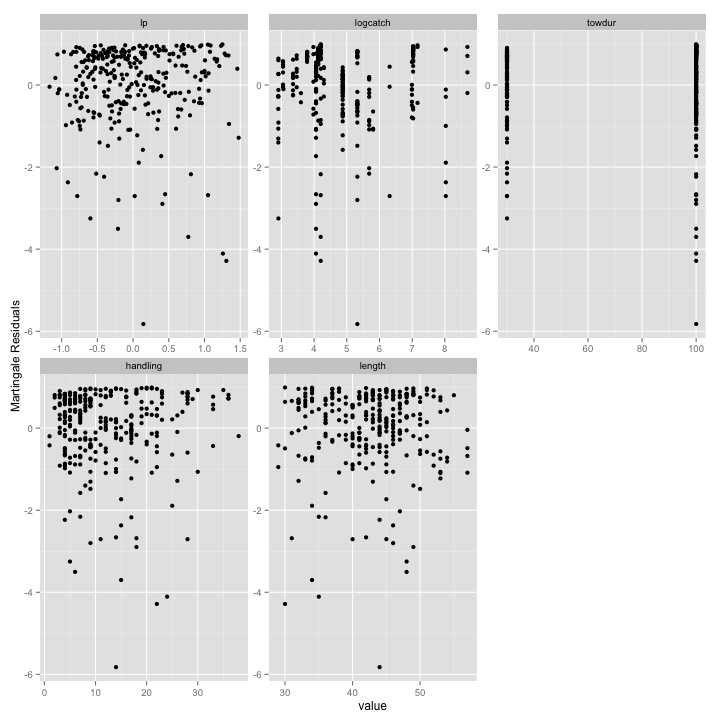## Deviance residuals It is a symmetric version of Martingale residuals. It is defined as a function of the Martingale residuals. $$\hat{D}_i = sign(\hat{r}_i) \sqrt{ - 2[\hat{r}_i + \delta_i log(\delta_i - \hat{r}_i)]}$$ ## Deviance residuals fish$dev <- residuals(res.coxph, type = "deviance")

## Melt dataset
fish.melt2 <- melt(fish, value.name = "value", id.vars = c("id","dev"),
measure.vars = c("lp","logcatch","towdur","handling","length"))

## Plot Martingale residuals against linear predictor and covariate
ggplot(data = fish.melt2, mapping = aes(x = value, y = dev)) +
layer(geom = "point") +
facet_wrap( ~ variable, scales = "free") +
scale_y_continuous(name = "Deviance Residuals")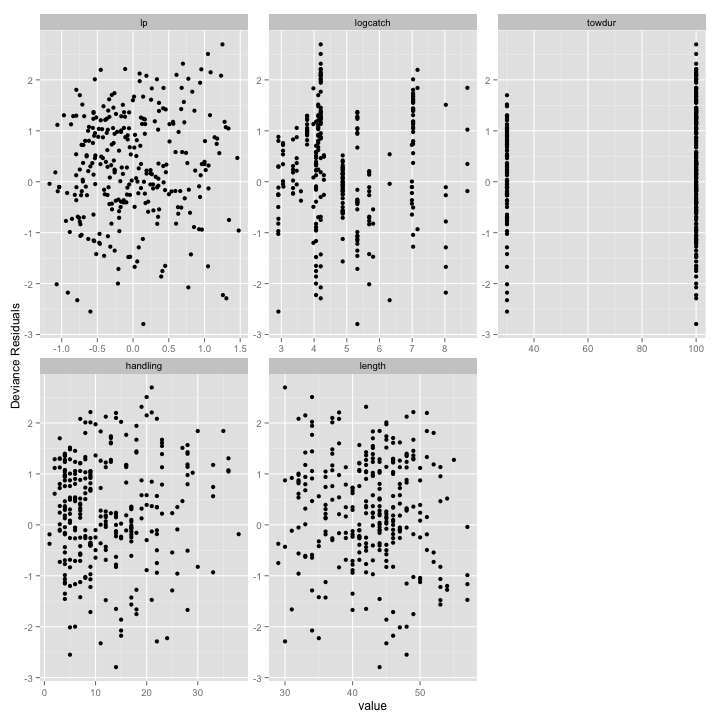## Schoenfeld residuals

These are defined at each observed failure time as:

$$r^s_{ij} = Z_{ij}(t_i) - \bar{Z}_j(t_i)$$

• calcuated for each covariate
• Represent the difference between the observed covariate and the average over the risk set at that time
• not defined for censored failure times (only for those who had events)
• useful for assessing time trend or lack or proportionality, based on plotting versus event time
• sum to zero, have expected value zero, and are uncorrelated in large samples
## Schoenfeld residuals (defined for each variable for uncensored subjects)
resid.schoenfeld <- residuals(res.coxph, type = "schoenfeld")

## Extract survival times (rownames)
survtime <- as.numeric(rownames(resid.schoenfeld))

## Transform to a data frame
resid.schoenfeld <- data.frame(resid.schoenfeld, row.names = NULL)

resid.schoenfeld$survtime <- survtime ## Melt resid.schoenfeld.melt <- melt(resid.schoenfeld, id.vars = c("survtime")) ## Plot ggplot(data = resid.schoenfeld.melt, mapping = aes(x = survtime, y = value)) + layer(geom = "point") + facet_wrap( ~ variable, scales = "free") + scale_y_continuous(name = "Schoenfeld residuals")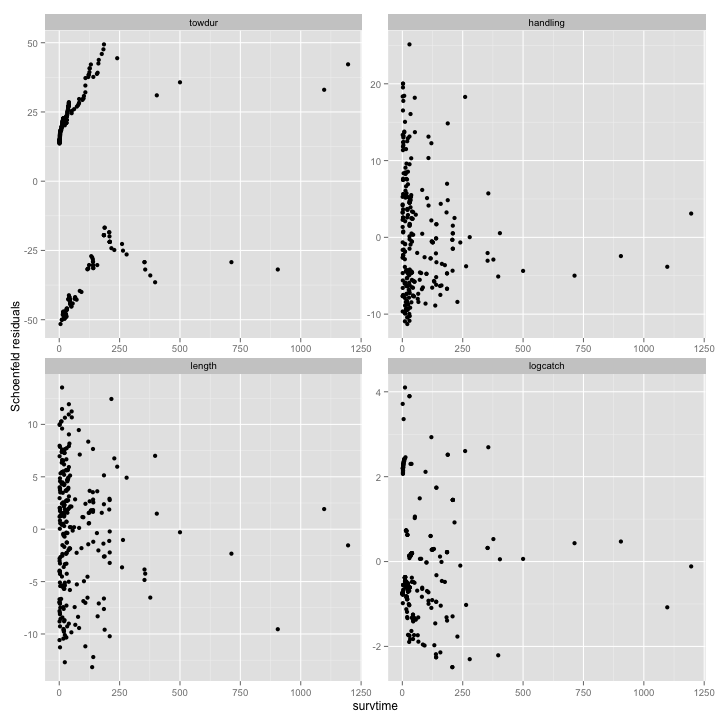## Weighted Schoenfeld residuals These are used more often than the unweighted version, as these are more like the typical OLS residuals (i.e., symmetric around 0). These are defined as: $$r^w_{ij} = n \hat{V} r^s_{ij}$$ where $$\hat{V}$$ is the estimated variance of $$\hat{\beta}$$. The weighted residuals can be used in the same way as the unweighted ones to assess time trends and lack of proportionality. ## Weighted Schoenfeld residuals (defined for each variable for uncensored subjects) resid.wt.schoenfeld <- residuals(res.coxph, type = "scaledsch") ## Extract survival times (rownames) survtime <- as.numeric(rownames(resid.wt.schoenfeld)) ## Transform to a data frame resid.wt.schoenfeld <- data.frame(resid.wt.schoenfeld, row.names = NULL) ## Name variables (somehow not done with scaledsch) names(resid.wt.schoenfeld) <- names(coef(res.coxph)) ## Add survival time resid.wt.schoenfeld$survtime <- survtime

## Melt
resid.wt.schoenfeld.melt <- melt(resid.wt.schoenfeld, id.vars = c("survtime"))

## Plot
ggplot(data = resid.wt.schoenfeld.melt, mapping = aes(x = survtime, y = value)) +
layer(geom = "point") +
facet_wrap( ~ variable, scales = "free") +
scale_y_continuous(name = "Weighted Schoenfeld residuals")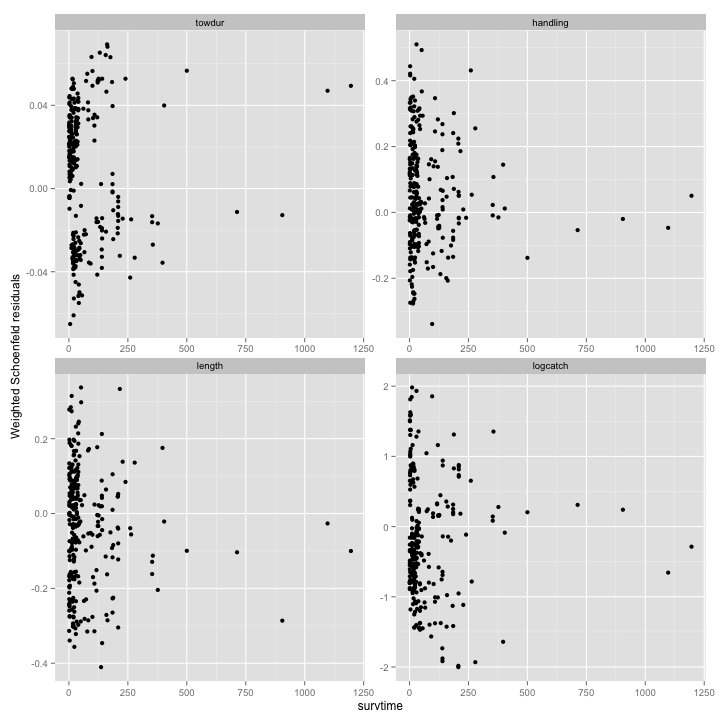## Deletion diagnostics

dfbeta and standardized version dfbetas are defined for each observation and for each of the predictors.

## dfbeta

         [,1]        [,2]        [,3]      [,4]
1  0.00006059  0.00025129 -0.00014247 -0.002683
2  0.00004993  0.00018484 -0.00019319 -0.001987
3  0.00005245  0.00015740 -0.00022945 -0.001932
4  0.00010402  0.00045771  0.00046060 -0.005432
5 -0.00007135 -0.00006347 -0.00007234  0.003060
6  0.00008616  0.00020294 -0.00018309 -0.003334


## dfbetas

      [,1]      [,2]      [,3]     [,4]
1  0.03004  0.025459 -0.014207 -0.05263
2  0.02476  0.018727 -0.019264 -0.03897
3  0.02601  0.015947 -0.022880 -0.03790
4  0.05157  0.046372  0.045930 -0.10654
5 -0.03537 -0.006431 -0.007213  0.06002
6  0.04272  0.020561 -0.018258 -0.06539


# Assessing the proportional hazards assumption

## Approaches for checking the PH assumption

• I Graphical
• (a) Plots of survival estimates for two groups, using KM estimates (marginal)
• (b) Plots of log[-log($$\hat{S}$$)] vs log(t) for two or more subgroups, using KM estimates (marginal)
• (c.) Plots of observed survival probabilities versus expected under PH model (Kleinbaum ch 4)
• (d) Plots of weighted Schoenfeld residuals from Cox models vs time

• II Use of goodness of fit test

• III Including interaction terms between a covariate and time

## Load foreign package for Stata data
library(foreign)



## (a) Plots of survival estimates for two groups, using KM estimates (marginal)

## Create survival object
nhome\$SurvObj <- with(nhome, Surv(los,cens == 1))

## Grouped by gender
LOSbyGender <- survfit(SurvObj ~ gender, data = nhome)
plot(LOSbyGender)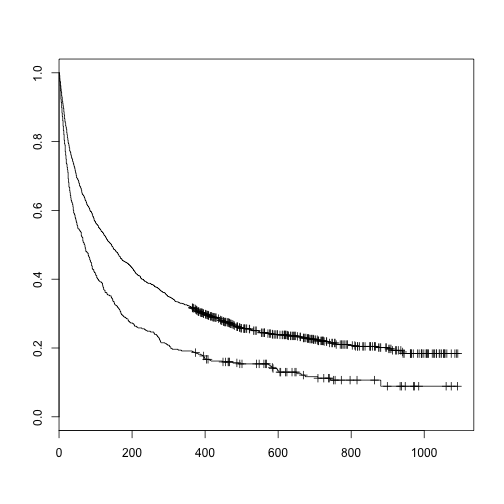## Grouped by married
LOSbyMarried <- survfit(SurvObj ~ married, data = nhome)
plot(LOSbyMarried)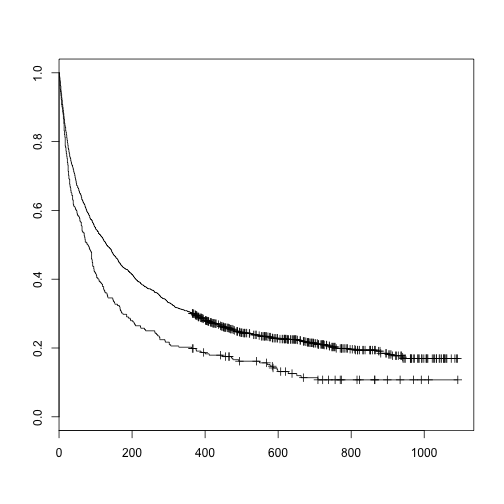## Grouped by health status (2,5 only) and gender
LOSbyHealthGender <- survfit(SurvObj ~ health + gender, data = nhome,
subset = health %in% c(2,5))
plot(LOSbyHealthGender)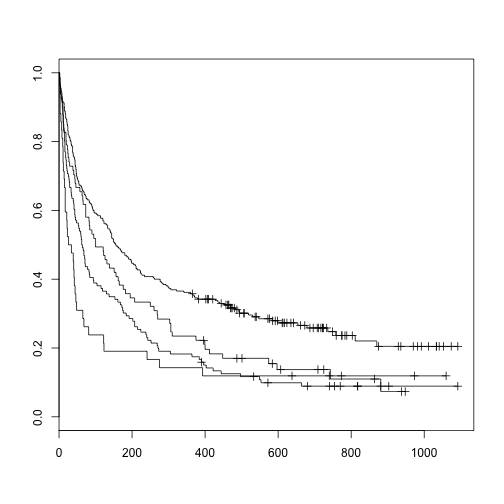## (b) Plots of log[-log($$\hat{S}$$)] vs log(t) for two or more subgroups, using KM estimates (marginal)

## by gender
with(LOSbyGender, plot(log(-log(surv)) ~ log(time)))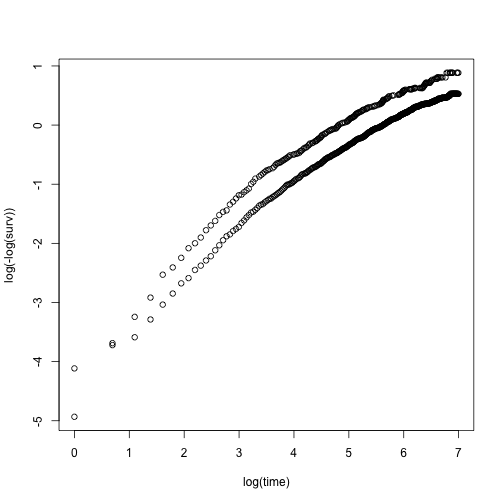## by gender, using survival::plot.survfit()
plot(LOSbyGender, fun = function(S) log(-log(S)), log = "x")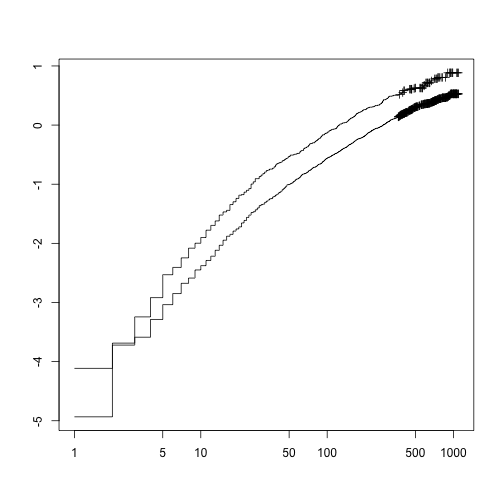## by gender, using rms::survplot()
library(rms)
survplot(fit  = LOSbyGender,
xlim = c(0,7),
conf = c("none","bands","bars"),
xlab = "",
label.curves = list(keys = "lines"),  # legend instead of direct label

## Create log(-log(S(t))) by log(time) plot
loglog   = TRUE,                         ## log(-log Survival) plot
logt     = TRUE,                         ## log time
)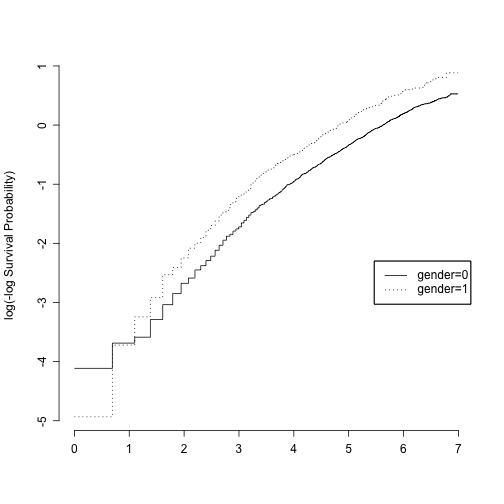## by marital status, using rms::survplot()
survplot(fit  = LOSbyMarried,
xlim = c(0,7),
conf = c("none","bands","bars"),
xlab = "",
label.curves = list(keys = "lines"),  # legend instead of direct label
## Create log(-log(S(t))) by log(time) plot
loglog   = TRUE,                         # log(-log Survival) plot
logt     = TRUE,                         # log time
)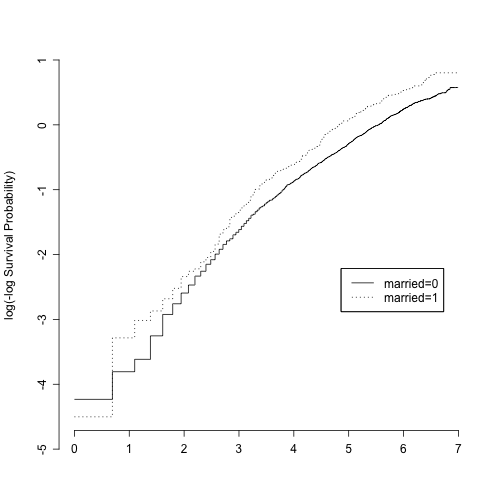## More than two groups, using rms::survplot()
survplot(fit  = LOSbyHealthGender,
xlim = c(0,7),
conf = c("none","bands","bars"),
xlab = "",
label.curves = list(keys = "lines"),  # legend instead of direct label
## Create log(-log(S(t))) by log(time) plot
loglog   = TRUE,                        # log(-log Survival) plot
logt     = TRUE,                        # log time
)